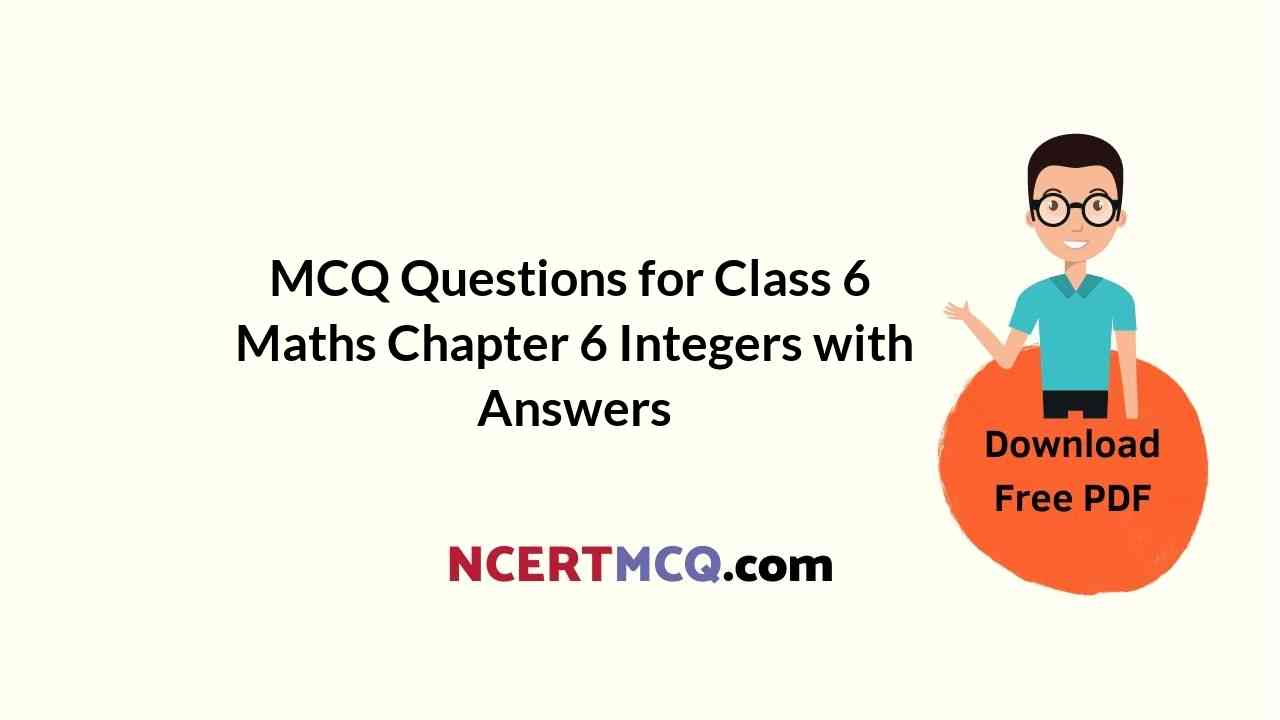Check the below NCERT MCQ Questions for Class 6 Maths Chapter 6 Integers with Answers Pdf free download. MCQ Questions for Class 6 Maths with Answers were prepared based on the latest exam pattern. We have provided Integers Class 6 Maths MCQs Questions with Answers to help students understand the concept very well.

Students can also refer to NCERT Solutions for Class 6 Maths Chapter 6 Integers for better exam preparation and score more marks.

## Integers Class 6 MCQs Questions with Answers

Integers Class 6 MCQ Chapter 6 Question 1.
The succeeding number of the number 0 is
(a) – 1
(b) 0
(c) 1
(d) 11.

MCQ On Integers For Class 6 Chapter 6 Question 2.
The succeeding number of the number – 1 is
(a) – 1
(b) 1
(c) 0
(d) -11.

Integers MCQ Class 6 Chapter 6 Question 3.
The succeeding number of the number – 4 is
(a) – 1
(b) – 2
(c) -3
(d) -4

Class 6 Maths Chapter 6 MCQ  Question 4.
The succeeding number of the number 9 is
(a) 9
(b) 8
(c) 10
(d) 11.

MCQ Questions For Class 6 Maths Integers Question 5.
The preceding number of the number 1 is
(a) 1
(b) – 1
(c) 2
(d) 0.

Class 6 Integers MCQ Chapter 6 Question 6.
The preceding number of the number 0 is
(a) 1
(b) – 1
(c) 0
(d) none of these.

MCQ Questions For Class 6 Maths Chapter 6 Question 7.
The preceding number of the number 2 is
(a) 3
(b) 1
(c) 0
(d) 4

Integers Class 6 Worksheet MCQ Chapter 6 Question 8.
The preceding number of the number 6 is
(a) 2
(b) 3
(c) 4
(d) 5

MCQ Questions On Integers For Class 6 Question 9.
An integer between – 3 and – 1 is
(a) – 3
(b) – 1
(c) – 2
(d) 0

Class 6 Maths Integers MCQ Chapter 6 Question 10.
Which of the following is true?
(a) 0 < – 8
(b) 0 > – 8
(c) 4 < – 4
(d) 0 > 6.

MCQ Integers Class 6 Chapter 6 Question 11.
Which of the following is false?
(a) – 1 < – 2
(b) 79 < 89
(c) – 1 < 1
(d) 1 > 0.

MCQ Of Integers For Class 6 Chapter 6 Question 12.
Which of the following statements is true?
(a) Every positive integer is larger than every negative integer.
(b) Zero is greater than every positive integer.
(c) Zero is smaller than every negative integer.
(d) Farther a number from zero to the right, smaller is its value.

MCQ Of Integers Class 6 Chapter 6 Question 13.
Which of the following statements, is false?
(a) Zero is neither a negative integer nor a positive integer.
(b) Zero is less than every positive integer.
(c) Zero is larger than every negative integer.
(d) Farther a number from zero on the left, larger is its value.

Ncert Class 6 Maths Chapter 6 MCQ Question 14.
Which of the following statements, is true?
(a) Greatest negative integer is – 1.
(b) – 10 is to the right of – 8 on a number line.
(c) – 50 is to the left of – 100 on a number line.
(d) – 11 is larger than – 10.

MCQ Class 6 Maths Chapter 6 Question 15.
(+ 1) + (+ 2) = ?
(a) + 1
(b) + 2
(c) + 3
(d) – 3

Question 16.
(- 1) + ( – 2) = ?
(a) – 1
(b) – 2
(c) – 3
(d) + 3

Question 17.
(- 2) + (+ 3) = ?
(a) – 2
(b) + 3
(c) + 1
(d) – 1

Question 18.
(- 4) + (+ 3) = ?
(a) – 1
(b) 1
(c) 0
(d) + 2.

Question 19.
(- 1) + (- 1) = ?
(a) – 1
(b) – 2
(c) 2
(d) 0

Question 20.
(- 1) + (+ 1 ) = ?
(a) – 1
(b) + 1
(c) 0
(d) none of these.

Question 21.
(-2) – (- 1) = ?
(a) – 1
(d) 1
(c) 0
(d) – 2

Question 22.
(- 1) – (- 2) = ?
(a) – 1
(b) 1
(c) 0
(d) none of these.

Question 23.
(- 1) + ? = 0
(a) + 1
(b) – 1
(c) 0
(d) none of these.

Question 24.
10 + ? = 0
(a) – 1
(b) – 10
(c) 0
(d) 1

Question 25.
9 + (- 9) = ?
(a) 9
(b) -9
(c) 1
(d) 0

Question 26.
(- 1) + ? = – 2
(a) 1
(b) – 1
(c) 0
(d) 2

Question 27.
? – 5 = – 5
(a) 0
(b) 5
(c) -5
(d) 1

Question 28.
? – 4 = – 2
(a) 1
(b) 2
(c) 3
(d) 4.

Question 29.
2 – (- 1) – 2 – (- 3) =
(a) 0
(b) 3
(c) 2
(d) 4

Question 30.
2 – (- 1) – (- 2)
(a) 3
(b) 4
(c) 2
(d) 5